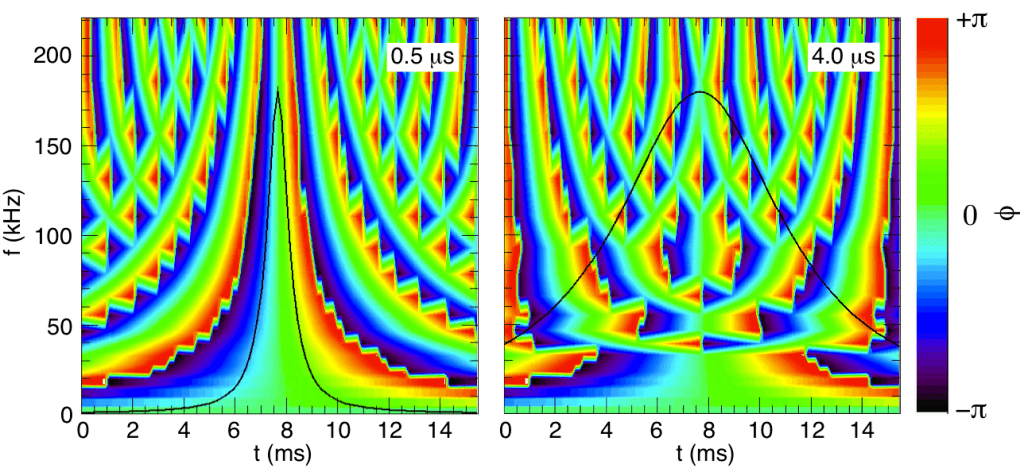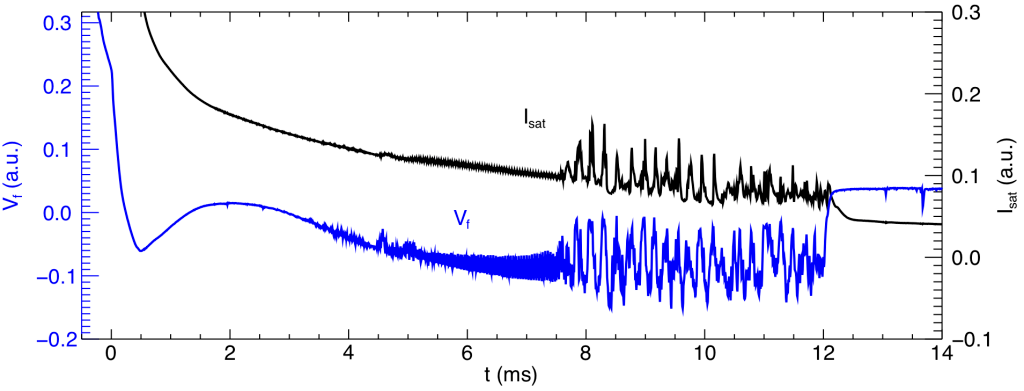# Thesis – Appendix B: Pulse Detection Techniques

### Pulse Detection Techniques

#### Overview

The study of exponential spectra and accompanying Lorentzian pulses in time series data requires that the pulses be isolated for determining their characteristic widths. A variety of identification techniques have been applied to the large data set of this experiment, and notes on two of these methods are provided here so that they may be further developed in future studies. The brute force method of sliding a Lorentzian shape through an entire time series is described in Section 4.3. The two other methods described here are the wavelet phase method in which constant phase over all frequencies identifies a pulse event, and the amplitude threshold method in which events of statistically significant amplitude are identified as pulses.

#### Wavelet Phase Method

Some aspects of wavelet transform techniques, including a comparison of their power spectra results to those of the more familiar Fourier methods, are discussed in Appendix A. The phase of a time series as calculated with wavelet techniques can be used to identify pulses because any singularity exhibits a constant phase [Farge, 1992] over all frequencies. Lorentzian pulses in this experiment are not necessarily singularities, and those with larger time widths are less so than narrower pulses. In spite of this difficulty, the wavelet phase method still appears to identify pulses fairly well.

Using the CWT as given in Eq. (A.2), which is a complex valued function for the Morlet wavelet basis, the phase, φ, is determined from, (Eq. B.1),$\phi = \tan^{-1}\left(\frac{I(W)}{R(W)}\right)$

where I and R signify the imaginary and real parts of the CWT respectively. The value of the phase at any frequency ranges from -π to +π.

##### Test Lorentzian Pulses

Figure B.1 presents the phase contours of two test signal Lorentzian pulses. One of these is narrower than the other, τ = 0.5 μs compared to τ = 4 μs. Both contours clearly identify a pulse by the symmetry of the phase values. The narrower pulse of the left panel more accurately reproduces the behavior of a singularity and the phase contour is remarkably sharper than that of the wider pulse in the right panel.Figure B.1: Phase contours of test signal Lorentzian pulses. The left panel shows the well defined phase pattern of a narrow (τ = 0.5 μs, overplotted) Lorentzian pulse. The right panel shows that wider pulses (τ = 4 μs, overplotted) still exhibit symmetry about the event, but that constant phase as a function of frequency is not as evident as for the narrower pulse.

Phase as a function of frequency for test signal Lorentzian pulses is shown in Fig. B.2. It is observed that narrower pulses demonstrate a more constant phase across the range of frequencies returned.

Measurements of Lorentzian pulses show that they can be either positive or negative polarity. Figure B.3 compares the phases of test signal Lorentzian pulses for each polarity. Both phase contours exhibit symmetry about the center of the pulse, but the negative polarity pulse features a much narrower region of zero phase. This result suggests that negative polarity pulses can be accurately identified by the wavelet phase technique, and even if this proves prohibitively difficult it is a simple task to invert the raw signals and apply the standards developed to isolate positive polarity events.Figure B.3: The wavelet phase is shown for positive (left panel) and negative (right panel) Lorentzian pulses. In both panels the test signal Lorentzian features τ = 1 μs and is overplotted in solid black.

##### Application to Experimental Results

Figure B.4 presents a measured pulse and its corresponding wavelet phase representation. The measurement is made in the outer region at r = 0.55 cm and features a large amplitude positive polarity pulse. The phase contour demonstrates excellent symmetry across the time of t = 7.17 ms where the pulse event is centered. This particular pulse exhibits a phase relationship that indicates it is similar to a singularity.

##### Limitations of the Wavelet Phase Detection Technique

An immediately apparent limitation of this technique is that it identifies only singularities, not Lorentzian pulses. In a best case scenario, the wavelet phase could be examined to determine the most likely temporal locations of Lorentzian pulses. These events could be extracted and then passed through a secondary analysis to determine whether they have any particular shape. Such a procedure essentially employs the same curve fitting technique as described in Section 4.3. That method simultaneously identifies events and classifies them as Lorentzian or non-Lorentzian, removing the need for the initial wavelet phase calculation. It is possible that the wavelet phase is useful in selecting out events that are embedded in background coherent fluctuations. In this case, the phase may be better suited for identifying a non-coherent mode related pulse which can then be further studied to determine whether it is a Lorentzian. This overcomes a limitation to the curve fitting technique in that fitting errors may incorrectly identify parts of a coherent oscillation as a unique Lorentzian pulse.

#### Amplitude Threshold Method

The amplitude threshold method isolates pulse events (again, not necessarily Lorentzian pulses) that exhibit statistically significant large amplitude. This is sometimes called “conditional averaging” in cases where the large amplitude events serve as triggers that set a reference time for another phenomenon.

Figure B.5 presents two raw measurements from the same discharge but different spatial positions. The Isat trace is measured at (r,z) = (0.5, 192) cm and the Vf trace is measured at (r,z) = (0.35, 544) cm. With an axial separation of Δ z = 352 cm, the correlation between the turbulent pulse region on both traces is interesting. The amplitude threshold method of pulse detection is used to study such correlations by allowing for the generation of ensemble profiles from a moving probe (in this case, the Vf measuring probe) based on the occurrence of similar pulses measured with a fixed Isat probe.Figure B.5: Same shot time series highlighting the correlation between pulses separated in position. The Isat trace (solid black, top) is placed in the outer region and detects little other than pulses. The Vf trace (solid blue, bottom) is placed in the gradient region and measures both coherent modes and turbulent pulses.

The identification of pulse events begins by calculating the standard deviation (σ), or root-mean-square (RMS), of the fluctuations in a signal. Figure B.6 is a plot of both the fluctuating component of the Isat signal (originally seen in Fig. B.5) and the RMS level as computed by a sliding window. The sliding window computes the RMS level for a limited time range of the entire signal and then translates the center of the window to calculate subsequent values. In this example, the RMS trace is useful for determining the likely beginning of the turbulent regime of the system. The increase in fluctuation level just after t = 7 ms suggests some type of transition has occurred, though it does not necessarily indicate anything about the presence of Lorentzian pulses.

An RMS value calculated over the entire time series of a single discharge is used to search for pulses. From a computational perspective, once the threshold has been assigned, any points above that value are collected as possible pulse peaks. For any sets of points that occur in sequence (i.e., any continuous collection of values that are all above the threshold), only points that represent local maxima are returned. This allows for the detection of overlapping pulses so long as they are minimally distinct.

Figure B.7 shows spikes in the data of Figs. B.5 and B.6 as found by the threshold technique. In this example the threshold level is set at 2.0, meaning that only features extended greater than two standard deviations above the fluctuation level for the entire signal are kept.

Figure B.8 highlights a weakness of the threshold method. If the level is set too low, then common oscillatory features are likely to be branded as pulses. In Fig. B.8 this is seen near the time t = 8.085 ms. At that time position there is a small oscillation (similar to those seen in the vicinity of t = 8.12 ms) that results in a peak. While the true fluctuation amplitude of this peak is small, by riding on top of the larger oscillation it satisfies the detection criteria and is labeled a pulse peak. Other similar looking features within this time range may appear as peaks, but actually feature flat regions without a singular maxima. The vector representation of the plot trace may result in the appearance of sharp peaks when none are present. In order to minimize erroneous spike detection the threshold should be set as high as possible.

While the shape of the pulses is not determined by this method, it may be safely assumed that a confirmation of their shape can be coupled with this conditional analysis to begin a study of the transport caused by pulses. At the very least, this type of analysis helps to determine the amount of transport that leads to the generation of outer region pulses.